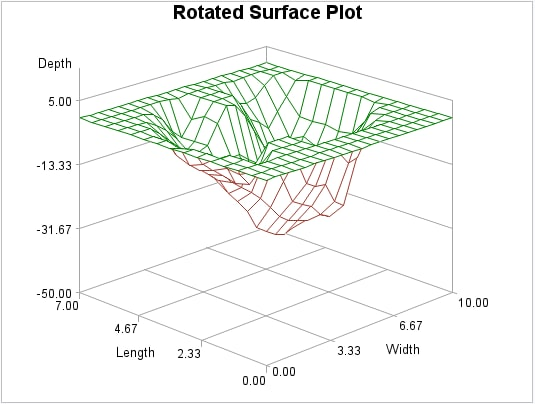# Example 2: Generating a Rotated Surface Plot

 Features: PLOT statement PLOT statement options: CTOP= GRID ROTATE= ZMAX= ZMIN= Data set: SASHELP.LAKE Sample library member: GTDROTAT
The surface plot shown in this example illustrates enhancements to the axes and the presentation. The plot illustrates a grid originating from the tick marks. A Z– axis range increase raised the plot above the horizontal X-Y plane. CTOP= green changed the top color and ROTATE= rotated the plot 45 degrees toward the viewer.## Program

`goptions reset=all border;`
`title "Rotated Surface Plot";`
```proc g3d data=sashelp.lake;
plot length*width=depth/
ctop=green
grid
rotate=45
zmax=5
zmin=-50;
run;
quit;```

## Program Description

Set the graphics environment.
`goptions reset=all border;`
Define the title.
`title "Rotated Surface Plot";`
Generate the surface plot.CTOP=green changes the color of the plot's top surface. The GRID option draws reference lines originating from the tick marks on all the axes. The ROTATE= option specifies a rotation angle of 45°. ZMAX=5 specifies the maximum value for the Z axis. ZMIN= –50 specifies the minimum value for the Z axis. Specifying a ZMIN= value that is below the minimum value in the input data set raises the plot above the horizontal plane. Data is not displayed if it exceeds the range specified by the ZMIN= and ZMAX= options.
```proc g3d data=sashelp.lake;
plot length*width=depth/
ctop=green
grid
rotate=45
zmax=5
zmin=-50;
run;
quit;```